## MP Board Class 12th Maths Important Questions Chapter 9 Differential Equations

### Differential Equations Important Questions

Differential Equations Objective Type Questions

Question 1.

Question 1.
Degree of the differential equation
$$\frac { d^{ 2 }y }{ d^{ 2 }x }$$ + x2 $$\frac{dy}{dx}$$ = ex is:
(a) 1
(b) 2
(c) 3
(d) does not exist
(c) 3

Question 2.
Solution of differential equation (1 + x) y dx + (1 – y) xdy = 0 is:
(a) log xy + x + y = c
(b) logxy + x – y = c
(c) log xy – x – y = c
(d) log xy – x + y = c.
(b) logxy + x – y = cQuestion 3.
The differential equation of all circles which passes through the origin whose center lie on the A – axis is:
(a) x2 = y2+ xy $$\frac{dy}{dx}$$
(b) x2 = y2 + 3xy $$\frac{dy}{dx}$$
(c) y2 = x2 + 2xy $$\frac{dy}{dx}$$
(d) y2 = x2 – 2xy $$\frac{dy}{dx}$$
(c) y2 = x2 + 2xy $$\frac{dy}{dx}$$

Question 4.
Solution of the differential equation $$\frac{dy}{dx}$$ + y = ex, y(o) = 0 is:
(a) y = e-x(x – 1)
(b) y = xex
(c) y = xe-x + 1
(d) y = xe-x
(d) y = xe-x

Question 5.
The straight line which satisfies the differential equation $$\frac{dy}{dx}$$ = m and cuts an intercept 3 on the positive y – axis is:
(a) y = mx + c
(b) y = mx +3
(c) y = mx – 3
(d) y = – mx + 3.
(b) y = mx +3

Question 2.
Fill in the blanks:

1. The corresponding differential equation of the equation x2 + y2 = a2 is ……………………………
2. The differential equation of the curve y = ecx is …………………….. where c is arbitrary constant.
3. The integration factor of the linear differential equation $$\frac{dy}{dx}$$ + Py = Q is …………………………….
4. In the linear differential equation $$\frac{dy}{dx}$$ + Py = Q. P and Q are …………………………….
5. The form of differential equation (x + y + 1) dy = dx is ……………………………
6. Solution of the differential equation e-x+y $$\frac{dy}{dx}$$ = 1 is ……………………………

1. y $$\frac{dy}{dx}$$ + x = 0
2. x $$\frac{dy}{dx}$$ = y log y
3. epdx
4. constant
5. linear differential equation
6. e-y = e-x + cQuestion 3.
Write True/False:

1. Order of differential equation y = x $$(\frac { dy }{ dx } )^{ 2 }$$ + $$\frac { d^{ 2 }y }{ dx^{ 2 } }$$ is 2.
2. Degree of differential equation $$(\frac { d^{ 3 }y }{ dx^{ 3 } } )^{ 4/5 }$$ – 2 $$(\frac { dy }{ dx } )$$ $$(\frac { d^{ 2 }y }{ dx^{ 2 } } )^{ 2 }$$ = 0 is 5.
3. The integration factor of linear differential equation x $$\frac{dy}{dx}$$ – y = 2x2 is e-x
4. Solution of differential equation dy = sin xdx is y + cos x – c = 0.
5. Solution of differential equation ydx + (x – y3) dy = 0 is xy = $$\frac { y^{ 4 } }{ 4 }$$ + c.

1. True
2. False
3. False
4. True
5. True

Question 4.
Write the answer is one word/sentence:

1. Write the integration factor of differential equation (1 + y2) + (2xy – cot y) $$\frac{dy}{dx}$$ = 0.
2. Find the solution of the differential equation (1 + x2) dy = (1 + y2) dx.
3. Find the sum of order and degree of the differential equation y = x $$(\frac { dy }{ dx } )^{ 3 }$$ + $$\frac { d^{ 2 }y }{ dx^{ 2 } }$$
4. Find the integration factor of the differential equation x logx $$\frac{dy}{dx}$$ + y = 2 log x
5. Find he solution of the differential equation $$\frac{dy}{dx}$$ + $$\frac{1}{x}$$ = $$\frac { e^{ y } }{ x^{ 2 } }$$

1. 1 + y2
2. x – y = c(1 + xy)
3. 3
4. log x
5. 2xe-y = cx2 + 1.

Differential Equations Very Short Answer Type Questions

Question 1.
Find the order and degree of $$\frac{dy}{dx}$$ + y = e-x?
1, 1

Question 2.
Find the degree and order of $$(\frac { dy }{ dx } )^{ 3 }$$ = $$\sqrt { 1+(\frac { dy }{ dx } )^{ 2 } }$$?
1, 6

Question 3.
Find the degree and order of $$\frac { d^{ 2 }y }{ dx^{ 2 } }$$ + $$\sqrt { 1+(\frac { dy }{ dx } )^{ 3 } }$$ = 0?
2, 2Question 4.
Find the differential equation corresponding to equation of circle x2 + y2 = a2?
y $$\frac{dy}{dx}$$ + x = 0

Question 5.
Find the differential equation of line y = mx + c?
$$\frac{dy}{dx}$$ = m

Question 6.
Solve the differential equation $$\frac{dy}{dx}$$ = 4y?
y = c.e4x

Question 7.
Solve the differential equation x2 $$\frac{dy}{dx}$$ = 2?
y = c – $$\frac{2}{x}$$Question 8.
Find the solution of differential equation dy = sinxdx?
y + cos x = c

Question 9.
Find the solution of differential equation $$\frac{dy}{dx}$$ + Px = Q?
xe∫pdy. dy + c

Question 10.
Solve the differential equation (1 – y2) $$\frac{dy}{dx}$$ + yx = ay?
$$\frac { 1 }{ \sqrt { 1-y^{ 2 } } }$$

Differential Equations Short Answer Type Questions

Question 1.
Solve the differential equation xlog xdy – ydx = 0?
Solution:
x log x dy – ydx = 0 (given)
⇒ x log x = ydx
⇒ $$\frac{1}{y}$$ $$\frac{dy}{dx}$$ = $$\frac{1}{xlogx}$$ dx
⇒ ∫$$\frac{1}{y}$$ dy = ∫$$\frac{1}{xlogx}$$ dx
⇒ log y = ∫$$\frac{1}{t}$$ dt , (let log x = t ⇒ $$\frac{1}{x}$$ dx = dt)
⇒ log y = log t + log c
⇒ log y = log log x + log c

Question 2.
Solve the differential equation $$\frac{dy}{dx}$$ = ex-y + x.e-y?
Solution:
$$\frac{dy}{dx}$$ = ex-y + x.e-y (given)
⇒ $$\frac{dy}{dx}$$ = e-y (ex + x)
⇒ eydy = (ex + x) dx
Integrating both sides,
∫eydy = ∫(ex + x) dx
ey = ex + $$\frac { x^{ 2 } }{ 2 }$$ + cQuestion 3.
Prove that solution of y = 4sin 3x is $$\frac { d^{ 2 }y }{ dx^{ 2 } }$$ + 9y = 0?
Solution:
y = 4 sin 3x (given)
Differentiating with respect to x,
∴$$\frac{dy}{dx}$$ = 12 cos 3x
Again differentiating with respect to x,
$$\frac { d^{ 2 }y }{ dx^{ 2 } }$$ = – 36 sin 3x = – 9 × 4 sin 3x
⇒ $$\frac { d^{ 2 }y }{ dx^{ 2 } }$$ = -9y, [from eqn.(1)]
⇒ $$\frac { d^{ 2 }y }{ dx^{ 2 } }$$ + 9y = 0. Proved.

Question 4.
Solve the differential equation $$\frac{dy}{dx}$$ = sec2 x + 2x?
Solution:
$$\frac{dy}{dx}$$ = sec x (sec x + tan x) (given)
⇒ dy = (sec2 x + sec x tan x ) dx
⇒ ∫dy = ∫sec2 dx + ∫sec x tan x dx
∴y = tan x + sec x + c.

Question 5.
Solve the differential equation $$\frac{dy}{dx}$$ = sec2 x + 3x2?
Solution:
$$\frac{dy}{dx}$$ = sec2 x + 3x2 (given)
⇒ dy = (sec2 x + 3x2) dx
⇒ ∫dy = ∫sec2 xdx + 3∫x2 dx
⇒ y = tan x + $$\frac { 3x^{ 3 } }{ 3 }$$ + c
⇒ y = tan x + x3 + c.

Question 6.
Solve the differential equation $$\frac{dy}{dx}$$ = sec2 x + 2x?
Solution:
Solve as Q.No . 5Question 7.
Solve the differential equation $$\frac{dy}{dx}$$ = (3x2 x + 2)?
Solution:
$$\frac{dy}{dx}$$ = (3x2 + 2) (given)
⇒ dy = (3x2 + 2) dx
⇒ ∫dy = ∫(3x2 + 2) dx
⇒ y = 3 × $$\frac { x^{ 3 } }{ 3 }$$ + 2x = c = x2 + 2x + c.

Question 8.
Solve the differential equation x2 $$\frac{dy}{dx}$$ = 2?
Solution:
x2 $$\frac{dy}{dx}$$ = 2 (given)
⇒ dy = 2.x-2 dx
⇒ ∫dy = 2∫x-2 dx
⇒ y = 2 $$(\frac { -1 }{ x } )$$ + c

Question 9.
Solve the differential equation $$\frac{dy}{dx}$$ = x3 + sin 4x?
Solution:
$$\frac{dy}{dx}$$ = x3 + sin 4x (given)
⇒ dy = (x3 + sin 4x) dx
⇒ ∫dy = ∫x3 dx + ∫sin 4x dx
⇒ y = $$\frac { x^{ 4 } }{ 4 }$$ + $$(\frac { -cos4x }{ 4 } )$$ + c
⇒ y = $$\frac { x^{ 4 } }{ 4 }$$ – $$\frac { -cos4x }{ 4 }$$ + c

Question 10.
Solve the differential equation $$\frac{dy}{dx}$$ + 2x = e3x?
Solution:
$$\frac{dy}{dx}$$ + 2x = e3x (given)
⇒ $$\frac{dy}{dx}$$ = e3x – 2x
⇒ dy = (e3x – 2x) dx
⇒ ∫dy = ∫3x dx – 2∫x dx
⇒ y = e3x. $$\frac{1}{3}$$ e3x – x2 + cQuestion 11.
Solve the differential equation $$\frac{dy}{dx}$$ = $$\frac { cos^{ 2 }y }{ sin^{ 2 }x }$$ (given)
⇒ $$\frac { 1 }{ cos^{ 2 }y }$$ dy = $$\frac { 1 }{ sin^{ 2 }x }$$ dx
⇒ sec2 ydy = cosec2 xdx
⇒ ∫sec2 ydy = ∫cosec2 xdx
⇒ tan y = – cot x + c

Question 12.
Solve the differential equation (x2 + 1) $$\frac{dy}{dx}$$ = 1?
Solution: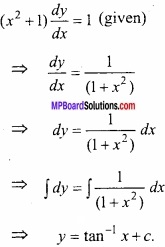Question 13.
Solve the differential equation $$\frac{dy}{dx}$$ = sin x sin y?
Solution:
$$\frac{dy}{dx}$$ = sin x sin y
⇒ cosec y dy = sin x dx
On integrating,
– loge (cosec y + cot y) = – cos x + c
⇒ cos x – loge (cosec y + cot y) = c

Question 14.
Solve the differential equation $$\frac{dy}{dx}$$ = y sin x?
Solution:
$$\frac{dy}{dx}$$ = y sin x
⇒ $$\frac{1}{y}$$ $$\frac{dy}{dx}$$ = sin x
⇒ ∫$$\frac{1}{y}$$ dy = ∫sin x dx
⇒ log y = – cos x + cQuestion 15.
Solve the differential equation $$\frac{dy}{dx}$$ = x cos x?
Solution:
Given:
$$\frac{dy}{dx}$$ = x cos x
⇒ dy = x cos x dx
⇒ ∫dy = ∫x cos x dx
⇒ y = xsin x – ∫1. sin x dx + c
⇒ y = xsin x + cos x + c

Question 16.
Solve the differential equation $$\frac{dy}{dx}$$ = x3 + sin 4x?
Solution:
$$\frac{dy}{dx}$$ = x3 + sin 4x (given)
⇒ dy = (x3 + sin 4x) dx
⇒ ∫dy = ∫x3 dx + ∫sin 4x dx
⇒ y = $$\frac { x^{ 4 } }{ 4 }$$ + $$(\frac { -cos4x }{ 4 } )$$ + c
⇒ y = $$\frac { x^{ 4 } }{ 4 }$$ – $$\frac { cos4x }{ 4 }$$ + c

Question 17.
Solve the differential equation $$\frac{dy}{dx}$$ + 2x = e3x?
Solution: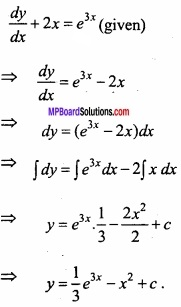Question 18.
Solve the differential equation $$\frac{dy}{dx}$$ = $$\frac { cos^{ 2 }y }{ sin^{ 2 }x }$$?
Solution:
$$\frac{dy}{dx}$$ = $$\frac { cos^{ 2 }y }{ sin^{ 2 }x }$$
⇒ $$\frac { 1 }{ cos^{ 2 }y }$$ dy = $$\frac { 1 }{ sin^{ 2 }x }$$ dx
⇒ sec2 ydy = cosec2 xdx
∴∫sec2 ydy = ∫cosec2 xdx + c
tan y = – cotx + c

Question 19.
Solve the differential equation $$\frac{dy}{dx}$$ = 1 – x + y – xy?
Solution:
$$\frac{dy}{dx}$$ = 1 – x + y – xy (given)
⇒ $$\frac{dy}{dx}$$ = (1 – x) + y (1 – x)
⇒ $$\frac{dy}{dx}$$ = (1 – x) (1 + y)
⇒ $$\frac{dy}{1+y}$$ = (1 – x) dx
⇒ ∫$$\frac{dy}{1+y}$$ = ∫(1 – x) dx
⇒ loge (1 + y) = x – $$\frac { x^{ 2 } }{ 2 }$$ + cQuestion 20.
Solve the differential equation $$\frac{dy}{dx}$$ = (1 + x) (1 + y2)?
Solution:
$$\frac{dy}{dx}$$ = (1 + x) (1 + y2) (given)
⇒ $$\frac { 1 }{ 1+y^{ 2 } }$$ dy = (1 + x) dx
Integrating both sides,
⇒ tan-1y = x + $$\frac { x^{ 2 } }{ 2 }$$ + c.

Question 21.
Solve the differential equation:
$$\frac{dy}{dx}$$ = cot2 x?
Solution:
$$\frac{dy}{dx}$$ = cot2 x (given)
⇒ dy = cot2 x
⇒ ∫dy = ∫cot2 x dx
⇒ y = ∫(cosec2 x – 1) dx
⇒ y = – cot x – x + c

Differential Equations Long Answer Type Questions – II

Question 1.
(A) Solve the differential equation $$\frac{dy}{dx}$$ + y tan x = sec x?
Solution:
$$\frac{dy}{dx}$$ + y tan x = sec x (given)
Comparing the equation with $$\frac{dy}{dx}$$ + Py = Q,
P = tan x, Q = sec x
∴L.F. = e∫P dx = etanx dx = elog secx
⇒ L.F. = sec x
Applying formula
y × (1.F.) = ∫Q × (I.F.) dx + c
⇒ ∫sec2 x dx + c
⇒ y sec x = tan x + c

(B) Solve the differential equation $$\frac{dy}{dx}$$ + y tan x = sin x?
Solution:
Solve as Q.No.1 (A).Question 2.
Solve the differential equation $$\frac{dy}{dx}$$ = $$\frac { \sqrt { 1-y^{ 2 } } }{ \sqrt { 1-x^{ 2 } } }$$?
Solution: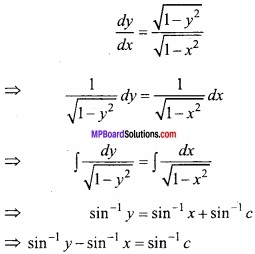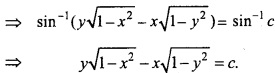Question 3.
Solve the differential equation 3x2 dy = (3xy + y2)dx?
Solution:
3x2 dy = (3xy + y2) dx (given)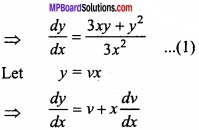Putting in eqn. (1),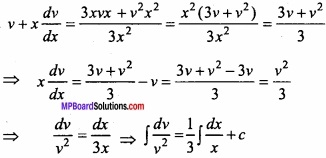⇒ – $$\frac{1}{v}$$ = $$\frac{1}{3}$$ log x + c
∴- $$\frac{1}{y}$$ = $$\frac{1}{3}$$ log x + c

Question 4.
Solve the differential equation (1 + x2) $$\frac{dy}{dx}$$ + 2xy = 4x2?
Solution: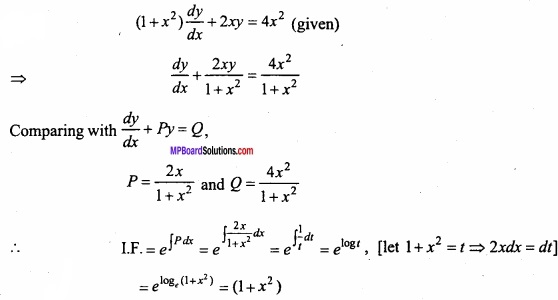The required solution will be: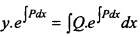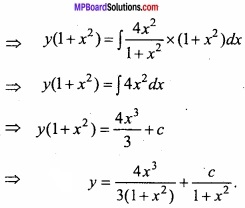Question 5.
Solve the differential equation (1 + x2) $$\frac{dy}{dx}$$ + 2xy = cos x?
Solution:
(1 + x2) $$\frac{dy}{dx}$$ + 2xy = cos x (given)
⇒ $$\frac{dy}{dx}$$ + $$\frac { 2x }{ (1+x^{ 2 }) }$$
Comparing with $$\frac{dy}{dx}$$ + Py = Q,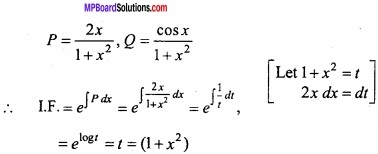The required solution of eqn. (1) is: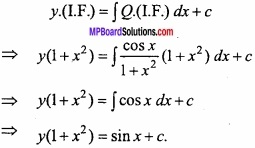Question 6.
Marginal cost price of making anything is given by equation c'(x) = $$\frac{dc}{dx}$$ = 2 + 0.15 x. Find the total cost price c(x) for making it? [Given c (0) = 100]
Solution:
c'(x) = $$\frac{dc}{dx}$$ = 2 + 0.15 x (given)
Integrating both sides
∫c'(x) dx = ∫ (2 + 0.15 x) dx
c(x) = 2x + 0.15 $$\frac { x^{ 2 } }{ 2 }$$ + A …………. (1)
If x = 0
c(0) = 2 × 0 + $$\frac { 0.15 }{ 2 }$$ × 02 + A
⇒ c(0) = A
∴ A = 100, [∵ c(0) = 100]
Putting in eqn. (1),
c(x) = 2x + 0.075 x2 + 100

Question 7.
Solve the differential equation x$$\sqrt { 1+y^{ 2 } }$$ dx + y $$\sqrt { 1+x^{ 2 } }$$ dy = 0?
Solution:
x$$\sqrt { 1+y^{ 2 } }$$ dx + y $$\sqrt { 1+x^{ 2 } }$$ dy = 0 (given)
⇒ $$\frac { y }{ \sqrt { 1+y^{ 2 } } }$$ dy = – $$\frac { x }{ \sqrt { 1+x^{ 2 } } }$$ dx
Integrating both sides,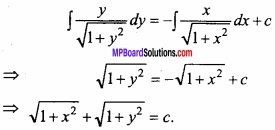Question 8.
Solve the differential equation (x + y + 1) $$\frac{dy}{dx}$$ = 1?
Solution:
(x + y + 1) $$\frac{dy}{dx}$$ = 1 (given)
⇒ $$\frac{dy}{dx}$$ = x + y + 1
⇒ $$\frac{dy}{dx}$$ – x = y + 1
Comparing the equation with $$\frac{dx}{dy}$$ + Px = Q?
P = – 1 and Q = y + 1
∴I.F. = epdy = e-∫dy = e-y
Here the required solution is:
⇒ x.epdy = ∫epdy. Qdy
⇒ x.e-y = ∫e-y (y + 1) dy + c
⇒ x.e-y = – (y + 1)e-y – ∫1(-e-y) dy + c
⇒ x = -(y + 1) -1 + cey
x + y + 2 = cey

Question 9.
Solve the differential equation sec2x tany dx + sec2 y tan xdy = 0?
Solution:
sec2x tany dx + sec2 y tan xdy = 0 (given)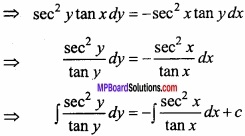⇒ log y = – log x + log c
⇒ log x + log y = log c
⇒ log xy = log c
⇒ xy = c

Question 10.
Solve the differential equation $$\frac{dy}{dx}$$ = y tanx – 2 sin x?
Solution:
$$\frac{dy}{dx}$$ – y tanx = – 2 sin x (given)
Comparing with $$\frac{dy}{dx}$$ + Py = Q,
P = – tan x, Q = – 2 sin x
L.F. = epdx = e-∫tanxdx
= elogecosx = cos x
The required solution is:
y.(I.F.) = ∫Q.I.F. dx + c
⇒ ycos x = -2∫sin x cos x dx + c
⇒ ycosx = -∫sin2xdx + c
⇒ ycosx = $$\frac { cos2x }{ 2 }$$ + c.Question 11.
Solve the differential equation $$\frac{dy}{dx}$$ + 2y = 4x?
Solution:
Solution:
$$\frac{dy}{dx}$$ + 2y = 4x
Comparing with $$\frac{dy}{dx}$$ + Py = Q,
P = 2, Q = 4x
I.F. = epdx = e∫2dx = e2x
The required differential solution is:
y. (I.F) = ∫Q.I.F. dx + c
⇒ ye2x = ∫4xe2x dx + c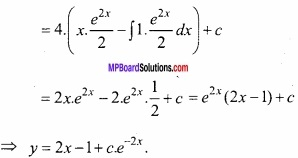Question 12.
Solve the differential equation cos2 x $$\frac{dy}{dx}$$ + y = 2?
Solution:
cos2 x $$\frac{dy}{dx}$$ + y = 2
⇒ $$\frac{dy}{dx}$$ + sec2 x. y = 2 sec2 x
Comparing with $$\frac{dy}{dx}$$ + P y = Q,
P = sec2 x, Q = 2 sec2 x
I.F. = e∫sec2 xdx
Required solution is:
y. (I.F) = ∫Q.I.F. dx + c
⇒ y.etanx = ∫2 sec2 x. etanx dx + c
⇒ y.etanx = 2.∫et dt + c, (Let tan x = t ⇒ sec2 xdx = dt)
= 2.etanx + c
⇒ etanx (y – 2) = c.Question 13.
Solve the differential equation cos x $$\frac{dy}{dx}$$ + y = sin x?
Solution:
cos x $$\frac{dy}{dx}$$ + y = sin x
⇒ $$\frac{dy}{dx}$$ + sec x.y = tan x
Comparing with $$\frac{dy}{dx}$$ + Py = Q,
P = secx, Q = tanx
L.F. = e∫secxdx
= eloge(sec x + tan x) dx + c
Required Solution y.I.F. = ∫Q.I.F. dx + c
⇒ y. (sec x + tan x) = ∫tan x.(sec x + tan x) dx + c
= ∫sec x tan x dx + ∫tan2 xdx + c
= sec x + ∫(sec2 x – 1) dx = sec x + tan x = – x + c.

Question 14.
Solve the differential equation (1 + y2) dx = (tan-1 y – x) dy? (CBSE 2015)
Solution:
(1 + y2) dx = (tan-1 y – x) dy (given)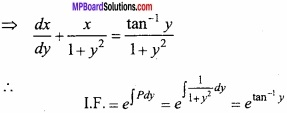The required solution is: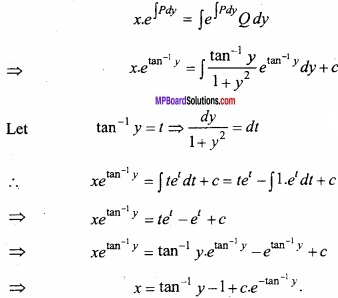Question 15.
Solve the differential equation (1 + y2) + ( x – etan-1 y) $$\frac{dy}{dx}$$ = 0? (CBSE 2016)
Solution:
The given equation is: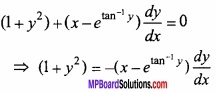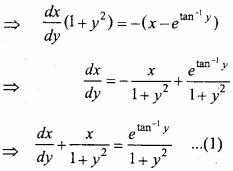Comparing with $$\frac{dx}{dy}$$ + Px = Q,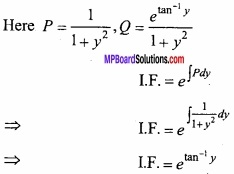The required solution is: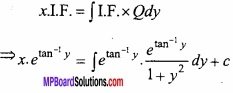Put tan-1 y = t,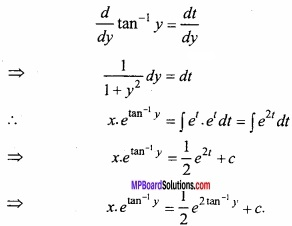Question 16.
Solve the differential equation (1 + x2) $$\frac{dy}{dx}$$ + 2xy = $$\frac { x }{ 1+x^{ 2 } }$$. Where, y = 0 and x = 1? (NCERT)
Solution: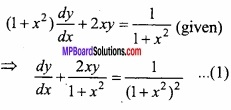Comparing with $$\frac{dy}{dx}$$ + Py = Q,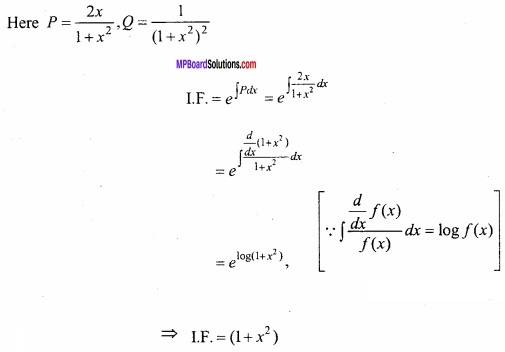The required solution is: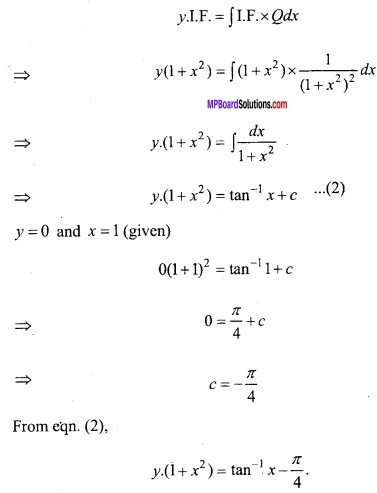y = 0 and x = 1 (given)
0 (1 + 1)2 = tan-1 1 + c
⇒ 0 = $$\frac { \pi }{ 4 }$$ + c
⇒ c = – $$\frac { \pi }{ 4 }$$
From eqn. (2),
y. (1 + x2) = tan-1 x – $$\frac { \pi }{ 4 }$$.Question 17.
Solve the differential equation $$\frac{dy}{dx}$$ + y cot x = 4x cosec x. Given: y = 0 and x = $$\frac { \pi }{ 2 }$$? (NCERT; CBSE, 2012)
Solution:
$$\frac{dy}{dx}$$ + y cot x = 4x cosec x (given)
Comparing with eqn. (1) $$\frac{dy}{dx}$$ + Py = Q,
P = cot x, Q = 4x cosec x
I.F. = epdx
= ecot xdx = elog(sinx) = sin x
The required solution is:
y.I.F = ∫I.F. × Qdx
⇒ y.sin x = ∫sin x × 4x cosec x dx
⇒ y sin x = 4∫$$\frac { xsinx }{ sinx }$$ dx
⇒ y sin x = 4∫xdx
⇒ ysin x = $$\frac { 4x^{ 2 } }{ 2 }$$ + c
⇒ y sin x = 2x2 + c
When x = $$\frac { \pi }{ 2 }$$ and y = 0,
0 (sin $$\frac { \pi }{ 2 }$$) = 2 ( $$\frac { \pi }{ 2 }$$ ) 2 + c
⇒ 0 = $$\frac { 2\pi ^{ 2 } }{ 4 }$$ + c
∴ c = $$\frac { -\pi ^{ 2 } }{ 2 }$$
Putting in eqn. (1),
y. sin x = 2x2 – $$\frac { -\pi ^{ 2 } }{ 2 }$$.